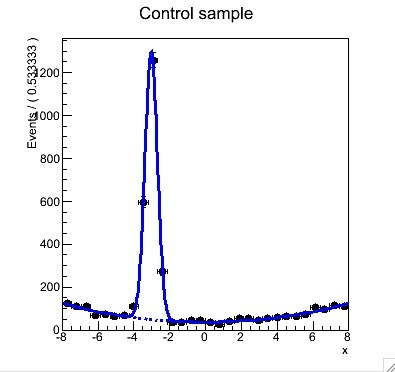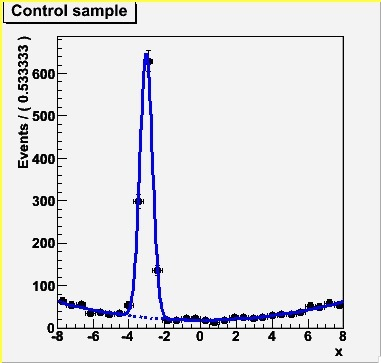# Different behavior by version?

Dear experts,

When I modified and tried to play with a tutorial rf501, I’ve found a very strange behavior of RooFit. ROOT version 5.26 gives the same result expected but ROOT version 5.28 and 5.30 give me different result that the histogram is somehow doubled as shown in the attached images.

What I did is only convert unbinned data to binned and import it as RooDataHist instead of RooDataSet.

``````// Generate 1000 events in x and y from model
RooDataSet *data = model.generate(RooArgSet(x),100) ;
RooDataSet *data_ctl = model_ctl.generate(RooArgSet(x),2000) ;

// Modified here - convert unbinned data to histogram
x.setBins(30);
TH1F* datah     = data->createHistogram("datah",x);
TH1F* datah_ctl = data_ctl->createHistogram("datah_ctl",x);

.........

// Import the data
RooDataHist combData("combData","combined data",x,Index(sample),Import("physics",*datah),Import("control",*datah_ctl)) ;``````

With unbinned data (as same as the tutorial rf501), the result is just the same but it seems to have some bug in importing binned data set. I used binary versions of ROOT for all on Fedora 12 and SLC5. I also attach the script what I used.

If this is a bug, it looks quite serious…
rf501_simultaneouspdf_hist.C (5.28 KB)